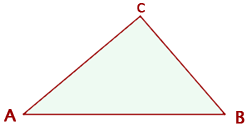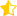Learn from home

The teachersA 3D vector is a line segment in three-dimensional space running from point A (tail) to point B (head).Each vector has a magnitude (or length) and direction.

### Components of a Vector

If the coordinates of A and B are: A(x1, y1, z1) and B(x2, y2, z2) the coordinates or components of the vectorare the coordinates of the head minus the coordinates of the tail.Calculate the components of the vectors that can be drawn in the triangle with vertices A(−3, 4, 0), B(3, 6, 3) and C(−1, 2, 1).### Magnitude or Length of a Vector

The magnitude of a vector is the length of the line segment that defines it.

The magnitude of a vector is always represented by a positive number and only the zero vector has a magnitude of zero.

## Calculation of the Magnitude to Know Its ComponentsGiven the vectorsand, find the magnitudes ofand·## Calculation of the Module Knowing the Coordinates of the Points### Distance between Two PointsFind the distance between the points A(1, 2, 3) and B(−1, 2, 0).### Unit Vector

The magnitude of the unit vector is one.

Normalizing a vector is obtaining another unit vector in the same direction.

To normalize a vector, divide it by its magnitude.Did you like the article?(6 votes, average: 3.33 out of 5)Loading...

Emma

I am passionate about travelling and currently live and work in Paris. I like to spend my time reading, gardening, running, learning languages and exploring new places.

Did you like
this resource?

Bravo!0 Followers

Most reacted commentRecent comment authors